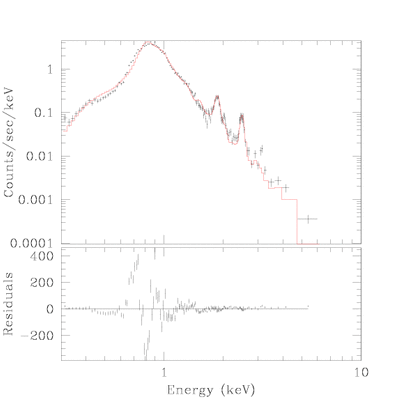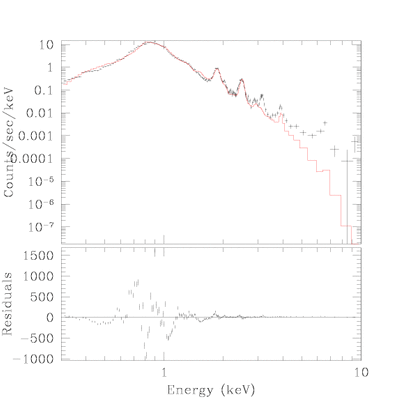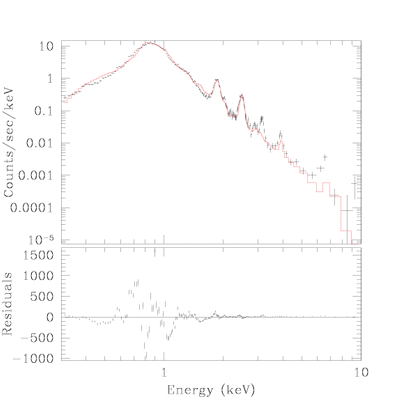True Color Image Gallery: Galactic SNR | Magellanic Cloud SNR

# Fit Detail for SNR J0519.5-6902

• nH was derived by fitting observed spectrum with two thermal plasma model.

## component A:

source=(xswabs * xsvraymond)
reduce chi^2 = 7.41398
nh = 0.2283 10^22/cm^2
PARAMETERS• DataSet 1: 153 data points -- 144 degrees of freedom.
• Statistic value = 1067.61
• Probability [Q-value] = 0
• Reduced statistic = 7.41398
--end--

## component B:

• dominant at outer region
• parameters were derived from clump at NE.
source=(xswabs * xsvraymond)
reduce chi^2 =
nh = 0.3298 10^22/cm^2
PARAMETERS• DataSet 3: 90 data points -- 76 degrees of freedom.
• Statistic value = 246.296
• Probability [Q-value] = 7.54433e-20
• Reduced statistic = 3.24074
--end--

## Total:

• Total spectrum was fiited with above two component.
• Only nH and normaliztion factor was thawed.
• Williams et al.(2001) derived nH=0.22 with vnpshock model.
source=(xswabs * (xsvraymond + xsvraymond))
reduce chi^2 =
nh = 0.2446 10^22/cm^2
PARAMETERS• DataSet 2: 200 data points -- 197 degrees of freedom.
• Statistic value = 3302.85
• Probability [Q-value] = 0
• Reduced statistic = 16.7657
--end--

## Total fit with additional component:

• For better estimation of flux
source=(xswabs * ((xsvraymond + xsvraymond) + xsraymond))
reduce chi^2 =
nh = 0.2446 10^22/cm^2
PARAMETERS• DataSet 2: 200 data points -- 199 degrees of freedom.
• Statistic value = 3227.76
• Probability [Q-value] = 0
• Reduced statistic = 16.2199
--end--

Back to SNR J0519.5-6902 IndexChandra Supernova Remnant CatalogMain Page | Galactic SNR | Magellanic Cloud SNRTrue Color Image Gallery: Galactic SNR | Magellanic Cloud SNR